C Program to Sort Word in String in Ascending Order

In this tutorial you will learn about the C Program to Sort Word in String in Ascending Order and its application with practical example.

C Program to sort Word in String in Ascending Order

In this tutorial, we will learn to create a C program that will sort Word in String in Ascending Order in C programming.

Prerequisites

Before starting with this tutorial we assume that you are best aware of the following C programming topics:

• Operators in C Programming.
• Basic Input and Output function in C Programming.
• Basic C programming.
• For loop in c programming.
• String functions of c programming.

Sort Word in String in Ascending Order

As we all know the String is a collection of character data types. In strings, only one variable is declared which can store multiple values. First, we will take the input string from the user.

Then we will sort that string using the for a loop.

With the help of this program, we can sort Word in String in Ascending Order.

Output:-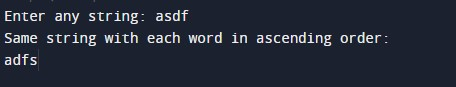In the above program, we have first initialized the required variable.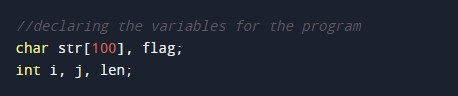• str = it will hold the string value.
• len = it will hold the integer value.
• i = it will hold the integer value.
• j = it will hold the integer value.
• flag = it will hold the string value.

Input strings from the user.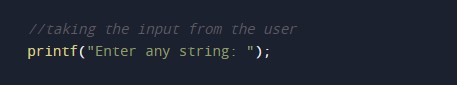Scanning string from the user.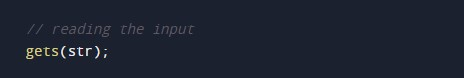Getting the length of the string.Sorting the string using for loop.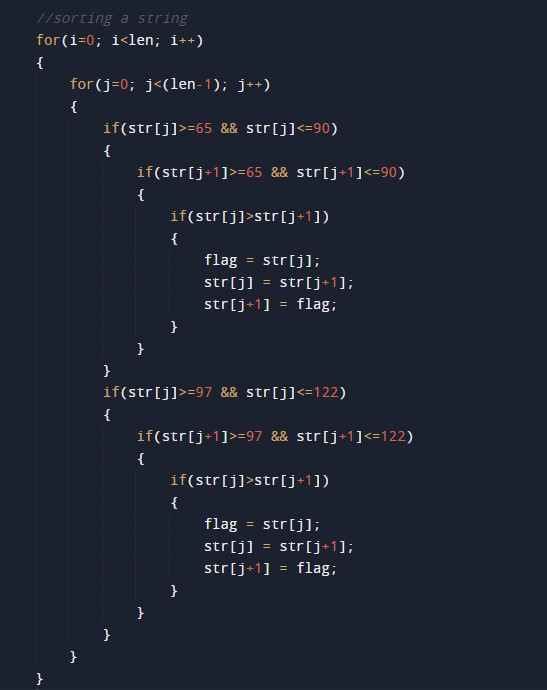Printing the output.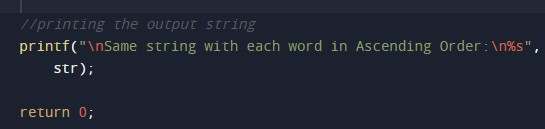In this tutorial we have learn about the C Program to Sort Word in String in Ascending Order and its application with practical example. I hope you will like this tutorial.